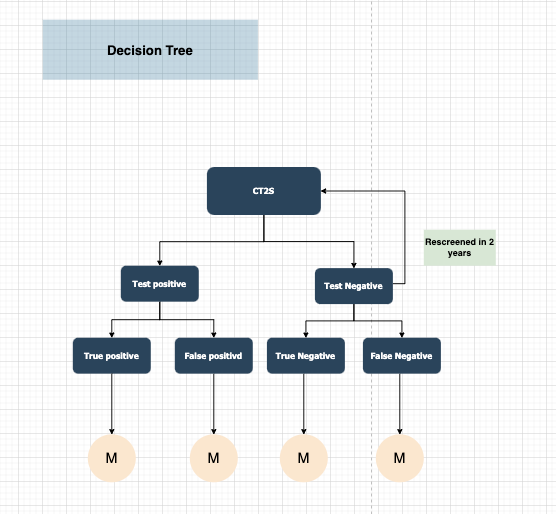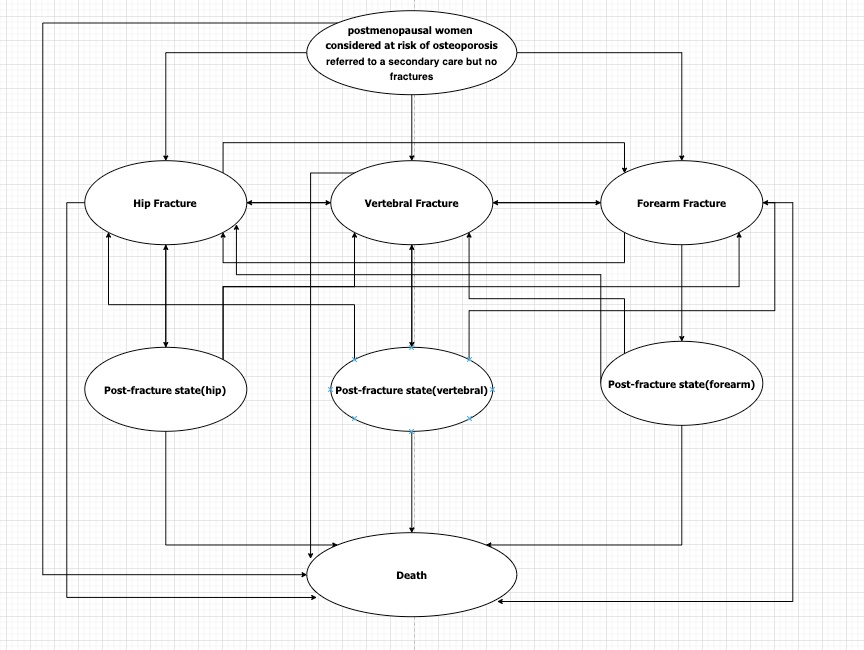# How to use TreeAge conduct cost effectiveness analysis for screening tools in rescreening scenerio

Hi

I am a researcher just start to use TreeAge recently. I'm trying to do a cost-effectiveness analysis for a new osteoporosis screening tool new decision tree + Markov model, the overall decision tree and followed Markov model is like this:(M in yellow circle mean corresponding Markov model)

However, one of the tricky parts is the screening need to be conducted every two years for the patients who are negative in the last screening and still in no fracture state, can I ask how can I use TreeAge to implement this rescreening strategy? Many thanks!

• This is a common question. The easiest way is to use the modulo function which does integer division like you learned in grade 2! 7 divide 5 goes once with two left over as a remainder. modulo(7;5) = 2. 7 divide 7 has no remainder, i.e modulo(7;7) = 0.

So let's say your cycle length is a month then you could create an expression for probability of screening in the Markov state for patients who are currently negative:

if(modulo(_stage;24)=0;1;0)

David

• Hi David,

Thanks! I will try it out!

best

Jieyi